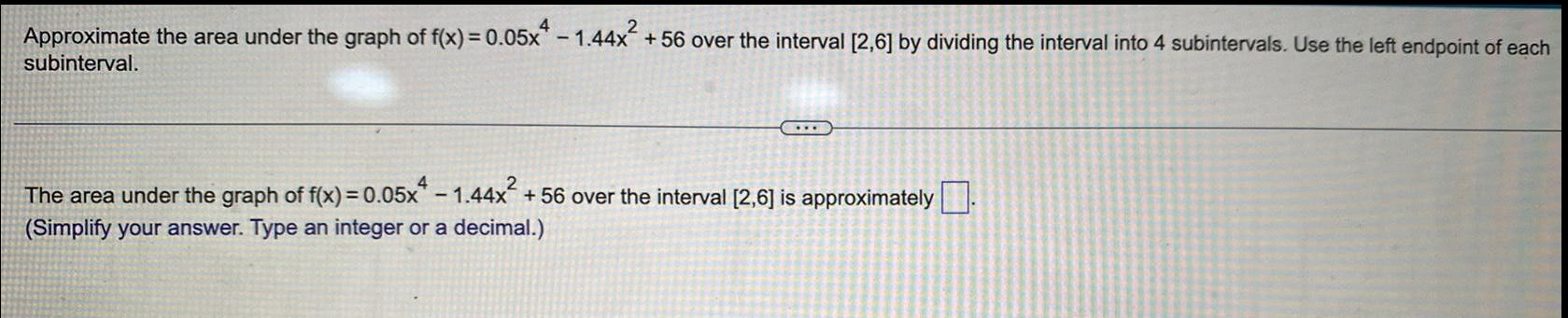Question:

# Approximate the area under the graph of f(x) = 0.05x -

Last updated: 8/14/2022Approximate the area under the graph of f(x) = 0.05x - 1.44x² +56 over the interval [2,6] by dividing the interval into 4 subintervals. Use the left endpoint of each subinterval. The area under the graph of f(x) = 0.05x - 1.44x² +56 over the interval [2,6] is approximately.